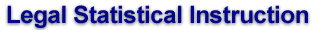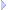Section1:2 x 2 TablesChi-SquareDegrees of Freedom

p-Value

Flip Coin 100 times
Breast Cancer Example

### Step 1-B: calculate degrees of freedom

To calculate the probability of observing the outcome by chance, one needs also to calculate the number of degrees of freedom.

When a comparison is made between one sample, with categories, and another sample, as in our coin flip example, a simple rule is that the degrees of freedom equal the product of (number of columns minus one) x (number of rows minus one). This calculation is done without counting the row and column containing the totals.

That is, (c-1) x (r-1).

 Number of heads Number of Tails Totals Coin A 5 5 10 Coin B 7 3 10 Totals 12 8 20

For the data above, this gives (2 - 1) x (2 - 1) = 1.

Another way of looking at this computation is to ask for the minimum number of figures that must be supplied, in addition to all the totals, that would allow us to complete the table. Here, any one figure in any of the four cells, along with the row and column totals, enables us to complete the table. Thus, there is one degree of freedom in this 2 x 2 table.

Copyright © 2002 by Theodore Eisenberg & Kevin M. ClermontCornell Law School Cornell University Comments to ted@teddy.law.cornell.edu Last updated: September 2002